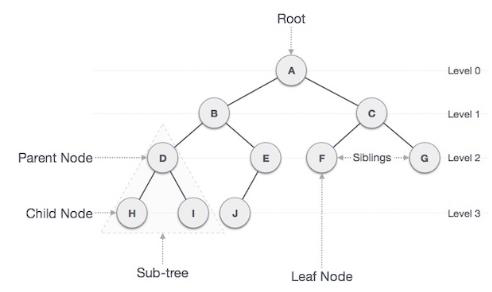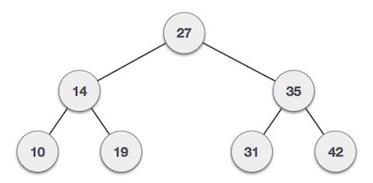# Count Non-Leaf nodes in a Binary Tree in C++

We are given with a binary tree and the task is to calculate the count of non-leaf nodes available in a binary tree.

Binary Tree is a special data structure used for data storage purposes. A binary tree has a special condition that each node can have a maximum of two children. A binary tree has the benefits of both an ordered array and a linked list as search is as quick as in a sorted array and insertion or deletion operation are as fast as in linked list. Non-leaf nodes are also known as parent nodes as they have more than 0 child and less than two children.

Structure of a binary tree is given below −## For example

InputOutput − count of non-leaf nodes is: 3

Explanation − In the given tree, we have 27, 14 and 35 as non-leaf nodes since they have more than 0 children and less than 2 children.

## Approach used in the below program is as follows

• Create the structure of a binary tree containing, pointer to a left node, pointer to a right node and a data part stored in a node

• Create a function that will insert a node whenever this function is called. For that, insert data in a new node and also set the right and left pointer of a new node to a NULL and return the node.

• Create a recursive function that will count the number of non-leaf nodes in a binary tree.

• Check If root is NULL or left of root is NULL and right of root is NULL then return 0
• Return 1 + recursive call to this function with left pointer + recursive call to this function with right pointer
• Print the count

## Example

Live Demo

#include <iostream>
using namespace std;
// Node's structure
struct Node {
int data;
struct Node* left;
struct Node* right;
};
// To define the new node
struct Node* newNode(int data){
struct Node* node = new Node;
node->data = data;
node->left = node->right = NULL;
return (node);
}
// Count the non leaf nodes.
int nonleaf(struct Node* root){
if (root == NULL || (root->left == NULL && root->right == NULL)){
return 0;
}
return 1 + nonleaf(root->left) + nonleaf(root->right);
}
// Main function
int main(){
struct Node* root = newNode(10);
root->left = newNode(21);
root->right = newNode(33);
root->left->left = newNode(48);
root->left->right = newNode(51);
cout << nonleaf(root);
return 0;
}

## Output

If we run the above code it will generate the following output −

count of non-leaf nodes is: 2